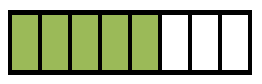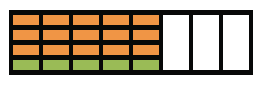### Home > CC1MN > Chapter 6 > Lesson 6.1.2 > Problem6-14

6-14.

Find each of the parts of parts described below. For each one, create a diagram that demonstrates your thinking.

1. $\frac { 3 } { 4 }$ of $\frac{5}{8}$

Draw a diagram to represent five eighths. A rectangle divided into 8 equal vertical sections, with the first 5 sections shaded.

Split five eighths into fourths. The same rectangle divided into 8 equal vertical sections. The first 5 sections are divided into four equal horizontal sections, with the first 3 rows of 5 rectangles shaded orange, and the last row of 5 rectangles shaded green.2. $\frac { 3 } { 8 } \cdot \frac { 2 } { 3 }$

Draw a diagram like you did for part (a). This will help you visualize the part of a fraction.

$\frac{6}{24}=\frac{1}{4}$

3. $\frac { 2 } { 3 }$ of $\frac { 7 } { 8 }$

Follow the steps in part (a).

4. $\frac { 4 } { 5 } \cdot \frac { 3 } { 7 }$

Follow the steps in part (a).